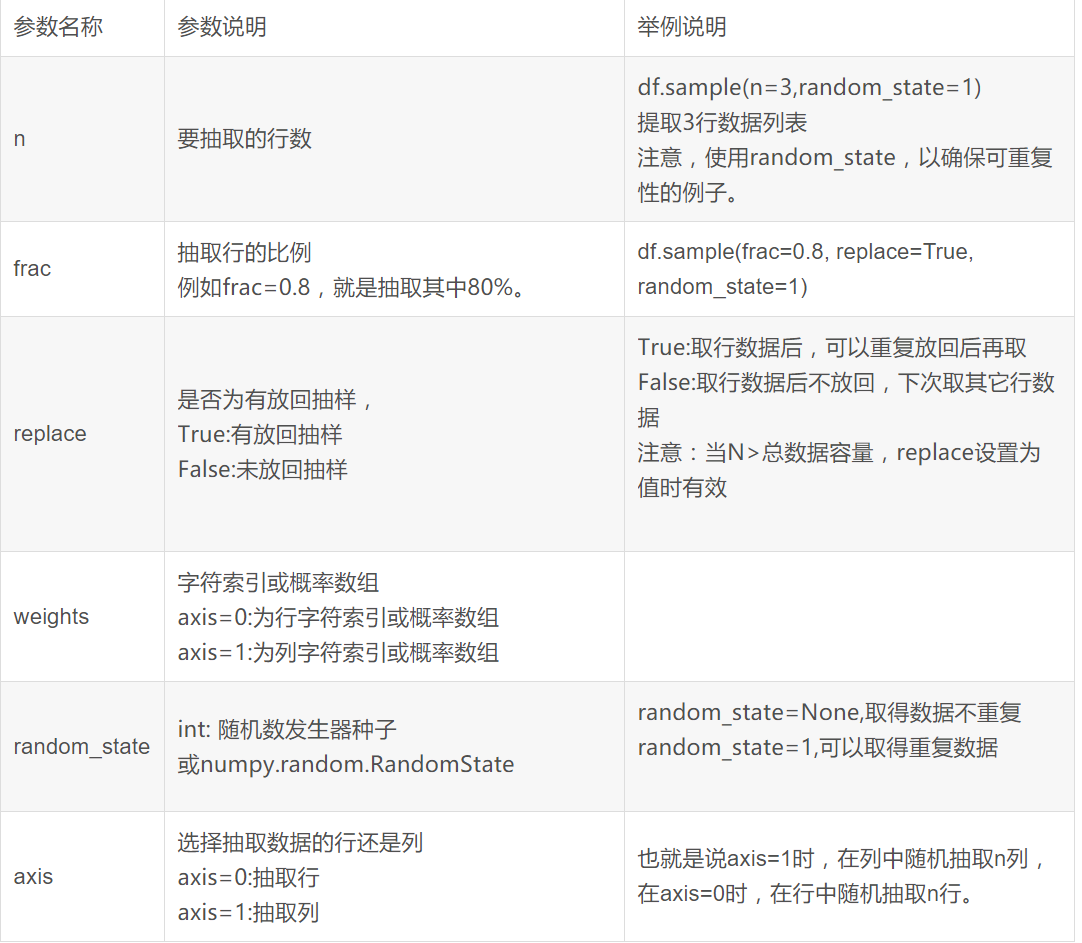# pandas小技巧------------df.sample随机抽样

https://www.cnblogs.com/webRobot/p/11484648.html

1、功能说明

DataFrame.sample(n=None, frac=None, replace=False, weights=None, random_state=None, axis=None)[source]

1. 输入参数说明n 要抽取的行数
df.sample(n=3,random_state=1)

frac

df.sample(frac=0.8, replace=True, random_state=1)
replace

True:有放回抽样
False:未放回抽样

True:取行数据后，可以重复放回后再取
False:取行数据后不放回，下次取其它行数据

weights

axis=0:为行字符索引或概率数组
axis=1:为列字符索引或概率数组

random_state
int: 随机数发生器种子

random_state=None,取得数据不重复
random_state=1,可以取得重复数据

axis

axis=0:抽取行
axis=1:抽取列

3. 返回值说明

01-02
02-23318104-27
05-028万+
05-267454
03-254547
02-152万+
04-169613
12-253506